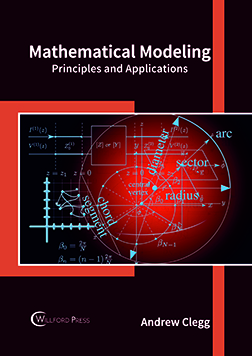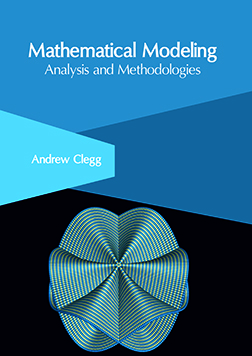BROWSE BY

#### Author

Results 1 - 2 of 2Mathematical Modeling: Principles and Applications Author : Andrew Clegg Subject : Engineering and Technology ISBN :9781682854167 Mathematical modeling is the method of developing mathematical models. This model is mainly based on mathematical language and theories and includes forms such as dynamical systems, statistical models, Read MoreMathematical Modeling: Analysis and Methodologies Author : Andrew Clegg Subject : Engineering & Technology ISBN :9781632406545 Mathematical models use mathematical tools to describe the performance and behavior of a system. They can be of different forms like statistical models, game theoretic models, dynamical systems, etc. Mathematical Read More
Results 1 - 2 of 2